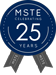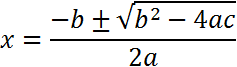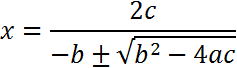MSTE
Office for Mathematics, Science, and Technology Education
College of Education @ University of Illinois## Description

When we have a quadratic function such as a*x2+b*x+c = 0, we can find the value of x by plugging the coefficients into a formula to solve the equation. This formula is called the quadratic formula:The quadratic formula is composed of a solution (x) and coefficients. The coefficients are the values of a, b, and c. Below is a variation of the quadratic formula:If you take a good look at both formulas, you will notice that the quadratic formula and its variation both contain b2-4ac. This is called the discriminant. The discriminant has the property of being an indicator for how many real roots are contained in the solution.

Arithmetic precision and whether or not rescaling has occurred affects the computational error of the quadratic formula. The web-based activity below was designed to aid in the exploration of finding the roots of a quadratic equation. The bottom window computes the roots using 15-17 digit precision and displays them rounded to the current selected precision.

## Simulation

Enter the coefficients to solve the equation ax2+bx+c=0:

Formula 1:
x=
-b ± √ b2-4ac
Over
2a
solution 1
Formula 2:
x=
2c
Over
-b ± √ b2-4ac
solution 2
Rounded Results

## Assessment Questions

• How many solutions do we have when b2-4ac > 0?
• How many solutions do we have when b2-4ac = 0?
• How many solutions do we have when b2-4ac < 0?
• What conclusions can we draw when summarizing the above findings?

Aria Live